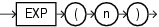## EXP

SyntaxDescription of the illustration exp.eps

Purpose

`EXP` returns `e` raised to the `n`th power, where `e` = 2.71828183... . The function returns a value of the same type as the argument.

This function takes as an argument any numeric data type or any nonnumeric data type that can be implicitly converted to a numeric data type. If the argument is `BINARY_FLOAT`, then the function returns `BINARY_DOUBLE`. Otherwise the function returns the same numeric data type as the argument.

The following example returns `e` to the 4th power:
```SELECT EXP(4) "e to the 4th power"